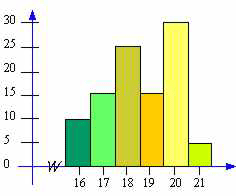### CFA Practice Question

There are 410 practice questions for this study session.

### CFA Practice Question

For the frequency distribution represented by the bar graph below, the range is ______.A. 16
B. 21
C. 6
Correct Answer: C

For a set of data, the range is the highest value minus the lowest value. For the data represented by the bar graph, the highest x-value is 21.5 and the lowest x-value is 15.5. So, the range is 21.5 - 15.5 (or 6). Note that if this bar graph represented a grouped frequency distribution, the range could not be found.

### User Contributed Comments5

User Comment
chhoda 16, 17, 18... are the midpts, therefore 21.5-15.5=6
bobert What is the grouped frequency distribution that is mentioned in the explanation?
bobert After reading the next question and its explanation it appears that a grouped freq distribution is simply one that has a range of numbers in its class. such as 12-19, 20-27, etc...
studyprep here each bar has at least 1 frequency and class width is also 1, so we can find the range. But if class width becomes greater than 1, than we can not find the range. also see the next question.
Sm7272 How do we know what element(s) actually existed there in the class 1 or the first bar. It could contain 10 elements of 16 , or 10 elements of 15.5. Definition of range is maximum value - minimum value. But how can we know what is the maximum data value from the graph ? Please clarify
You need to log in first to add your comment.